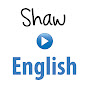# Practice English Speaking&Listening with: How to use the binocdf function on the TI - 84

Normal
(0)
Difficulty: 0

In this tutorial you will learn how to use the binomial

CDF function on the TI 84. For a binomial experiment

n stands for the fixed number trials, p stands for probability of success,

and r stands for the number of successes.

Let's consider this example: a surgical technique is performed on seven patients.

You are told there is a 70% chance of success.

Find the probability that the surgery is successful

for less than five patients, using technology.

The solution

shows that we first note

that the number of trials here n is 7

since the technique is performed on seven patients.

The probability of success is .70 since we're told there's a 70 percent

chance of success.

We were asked to find probability that the number sucesses is less

than five. This is also

finding the probability that there are no successes

plus one sucess plus 2 successes

plus 3 successes plus 4 successes.

The answer to this is: is

.353 or a 35.3 percent chance.

Let's find out how to find that answer using

the TI. To do this

we locate the "distribution" function on a TI.

We hit second "distribution"

and we scroll down until we find

binomial CDF. Notice that there's a binomial PDF that

is for exactly r successes, and the binomial CDF function

is for a less than statement involving

r, so less than or equal to r

number of successes. We select binomial CDF

and hit "enter" And we first enter

n, which is the number trials, in this problem we have 7.

We put a comma

and enter in the probability of success

in this problem we had .7,

next we hit comma, and we will enter in up to the number of succeses in this

case up to 4 (and including 4.)

So this function accumulates the probabilities starting at zero all the way up to and including

r success. In this case we want less than five

so we will use 4 as the

up to and including value. So we close the parentheses

and hit enter. Here we see the answer

is .35 and if you round to the third place value

you would use .353.

The Description of How to use the binocdf function on the TI - 84

### Channels for learning English

•English Academy
•ETJ English
•English Jade - Learn English (engVid)
•English with Joe
•Shaw English Online
•Speak English Now with Georgiana's mini-stories
•Real English With Real Teachers
•eJOY English